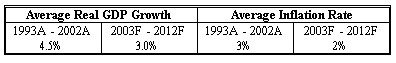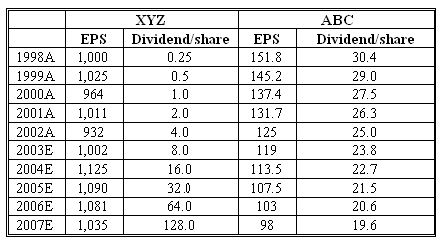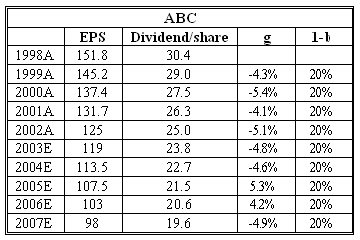### CFA Practice Question

There are 290 practice questions for this topic.

### CFA Practice Question

John is performing valuation of two companies - ABC and XYZ. Both companies have similar risk and the required rate of return is about 10%. John gathered the following data for his analysis:Determine the eligibility of the Gordon growth model for each of the two companies and estimate their values using the model.
Correct Answer: A simple observation of XYZ's earnings and dividends pattern shows that the Gordon growth model cannot be applied to this company. First of all, its dividends' growth (100% annually) by far exceeds the long-term sustainable growth of 5% (calculated as the sum of expected real GDP growth, 3%, and expected average inflation, 2%). Secondly, dividend payouts seem to be independent of the earnings pattern: XYZ's dividends double every year both in the past and during the forecast horizon, but its earnings are fairly constant around \$1,000. Therefore, we cannot assess the value of FBC using the Gordon growth model.ABC, on the other hand, is a perfect candidate for valuation based on the GGM. Both past and expected dividends fall at a stable rate of 4.75% (it can be estimated as (D2007/D2002)1/5 - 1 = (19.6/25.0)1/5 - 1 = -0.0475). Moreover, dividends constitute a fixed percentage of the company's earnings: 19.6 / 98 = 0.2 (20%). We can now calculate the value of ABC using the Gordon growth model: V2002 = D2002 (1 + g) / (r - g) = 25(1- 0.0475) / (0.10 + 0.0475) = \$161.44.

ABC has a negative dividend growth rate in infinity. Its earnings and value are also falling at a constant rate. Either the whole industry has gloomy prospects, or the company is slowly going out of business and its market share is consumed by other competitors.